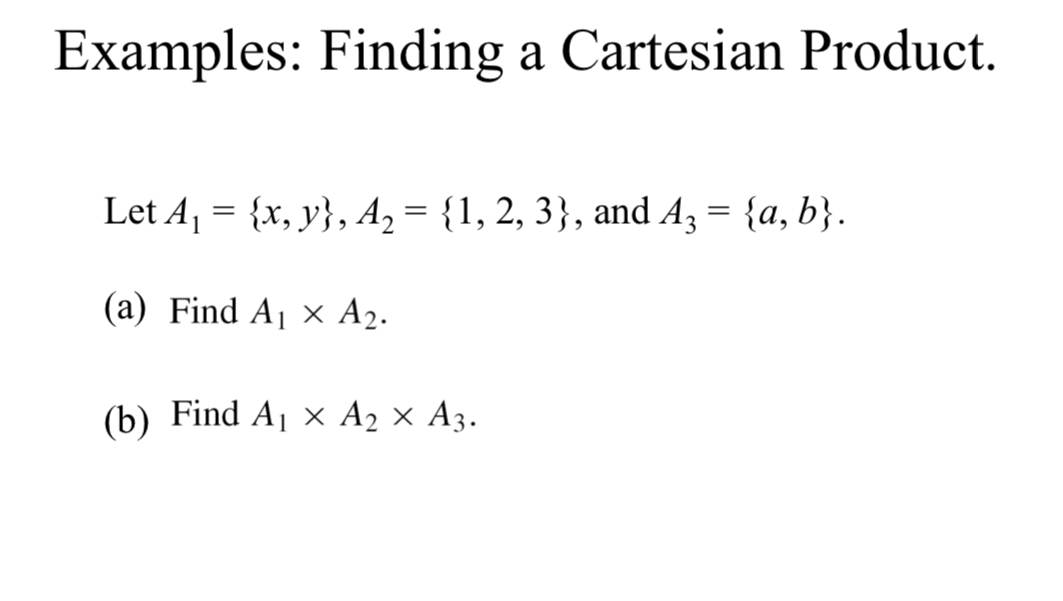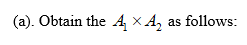# Examples: Finding a Cartesian ProductLet A1 {x, y, A2= {1, 2, 3}, and A3= {a, b}(a) Find A x A2.(b) Find A1 x A2 x A3.

Question
14 views

discrete mathhelp_outlineImage TranscriptioncloseExamples: Finding a Cartesian Product Let A1 {x, y, A2= {1, 2, 3}, and A3= {a, b} (a) Find A x A2. (b) Find A1 x A2 x A3. fullscreen
check_circle

Step 1

It is given that, the set is

Step 2...

### Want to see the full answer?

See Solution

#### Want to see this answer and more?

Solutions are written by subject experts who are available 24/7. Questions are typically answered within 1 hour.*

See Solution
*Response times may vary by subject and question.
Tagged in

### Math